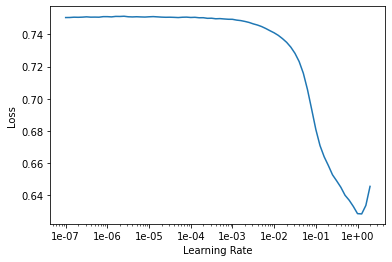# Low accuracy while trying to use ULMFit

Hi Everyone, using ULMFit for genre classification. Here I tried to do so using the movie `descriptions:`

```````learn = language_model_learner(data_lm,arch=AWD_LSTM,pretrained=True)`
`learn.lr` `_find(start_lr=1e-8, end_lr=1e2)`
`learn.recorder.plot()`
```````learn.fit_one_cycle(1,1e-2)`

accuracy = 0.31, which means that one-third of the time, the language model is predicting correctly. Moving forward:

``````learn.unfreeze()
learn.fit_one_cycle(cyc_len=5, max_lr=1e-2)
``````

accuracy remained at 0.31

`learn.save_encoder('ft_enc')`

Now, creating the classifier:

`data_clas = TextClasDataBunch.from_df('.',train_df=train_orig, valid_df=val_orig,text_cols='description',label_cols='genres',label_delim=',',vocab=data_lm.train_ds.vocab)`
`data_clas.show_batch()`
|xxbos xxmaj tom xxmaj xxunk is a police detective with a xxmaj christian sister , xxmaj eileen , a brother , xxmaj calvin , a wife , xxmaj susan , and eccentric brother - in - law , xxmaj jason . xxmaj one night , xxmaj jason seemingly goes insane and tries to kill xxmaj eileen , calling her a " xxunk " . xxmaj tom soon realises this may
|science fiction; thriller;drama|

As you can see, when creating the classifier data bunch, I am using the same vocab as the language model.

``````learn = text_classifier_learner(data_clas,arch=AWD_LSTM)
learn.freeze()
learn.lr` `_find(start_lr=1e-8, end_lr=1e2)
learn.recorder.plot()
````````````learn = text_classifier_learner(data_clas, arch=AWD_LSTM,metrics=[accuracy])
learn.freeze()
learn.fit_one_cycle(cyc_len=1, max_lr=1e-1, moms=(0.8, 0.7))
``````

accuracy = 0.01187

``````learn.lr_find()
learn.recorder.plot()
````````````learn.freeze_to(-2)
learn.fit_one_cycle(1, 1e-2, moms=(0.8,0.7))
``````

accuracy = 0.002

Can someone please tell me where I could be going wrong? Another basic question: what is the relationship between epoch and cyc_len? My understanding is that cyc_len = n means that for n epochs the learning rate will follow the increase-decrease pattern. Is that correct?